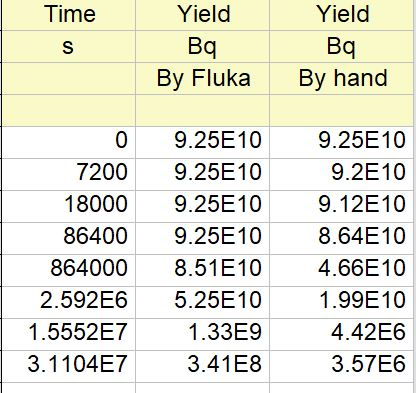# Where can I find the decay constant and cross section data in the Lib in Fluka

Dear experts,
I am so sorry to disturb you.
I met problem in the calculation with yield of 225Ac by proton + 232Th.
I calculated the yield of 225Ac by Fluka (irrdiate for 10days and then cooling), but the yield is quite strange. It may come up（or keep unchange） in the first several days and then decay after that (as shown in the Fig.1). So I calculated the yield of 225Ac hand by hand with the decay chain and their decay constants with formulus like solution for the bateman equation(You know, fluka solves the Bateman equation to find the result for the residual nucleus ). The results by hand is qute different from the result by Fluka. (I calculated many cases with fluka, the trend is almost the same. A simple case is 100MeV proton + 2mm depth 232Th, the beam intensity is 500uA.)

So I guess that the problem may exist in the decay constants or cross section data with these parent or daughter nucleus. Could you tell me where can I find them and how may this problem happen?

Best regards,

JiangfengDear Jiangfeng, it’s not clear how you obtained the numbers in the second column, since for the case you described I find the expected decaying behaviour instead. Please share your input file.

Dear Francesco Cerutti，
I am so sorry for the late reply. Here I attached a input file which produced similar result. Could you tell me where you found the expected decaying behaviour in the (Proton + Th target) simulation? Maybe I could learn from that.
The data from the second column is caculated by the theoretical solution with Betaman equation. We thought that it is better than the one from the Fluka, although Fluka solves the Betaman equation to find the result.